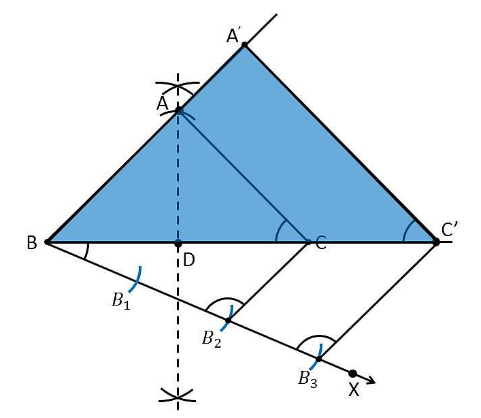Guru

# Construct an isosceles triangle whose base is 8cm and altitude 4cm and then another triangle whose sides are 1 whole 1/2 times the corresponding sides of the isoscles triangle. Q.4

• 0

What is the best way to solve this problem of question no 4 of class 10th ncert book of exercise 11.1 of constructions chapter, please give me the best and simple way to solve this problem Construct an isosceles triangle whose base is 8cm and altitude 4cm and then another triangle whose sides are 1 whole 1/2 times the corresponding sides of the isoscles triangle.

Share

1. Construction Procedure:

1. Draw a line segment BC with the measure of 8 cm.

2. Now draw the perpendicular bisector of the line segment BC and intersect at the point D

3. Take the point D as centre and draw an arc with the radius of 4 cm which intersect the perpendicular bisector at the point A

4. Now join the lines AB and AC and the triangle is the required triangle.

5. Draw a ray BX which makes an acute angle with the line BC on the side opposite to the vertex A.

6. Locate the 3 points B1, B2 and B3 on the ray BX such that BB1 = B1B2 = B2B3

7. Join the points B2C and draw a line from B3 which is parallel to the line B2C where it intersects the extended line segment BC at point C’.

8. Now, draw a line from C’ the extended line segment AC at A’ which is parallel to the line AC and it intersects to make a triangle.

9. Therefore, ΔA’BC’ is the required triangle.Justification:

The construction of the given problem can be justified by proving that

A’B = (3/2)AB

BC’ = (3/2)BC

A’C’= (3/2)AC

From the construction, we get A’C’ || AC

∴ ∠ A’C’B = ∠ACB (Corresponding angles)

In ΔA’BC’ and ΔABC,

∠B = ∠B (common)

∠A’BC’ = ∠ACB

∴ ΔA’BC’ ∼ ΔABC (From AA similarity criterion)

Therefore, A’B/AB = BC’/BC= A’C’/AC

Since the corresponding sides of the similar triangle are in the same ratio, it becomes

A’B/AB = BC’/BC= A’C’/AC = 3/2

Hence, justified.

• 0